## Example Questions

1 3 Next →

### Example Question #21 : How To Find The Equation Of A Perpendicular Line

Which of the following is NOT perpendicular to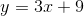?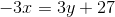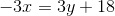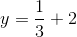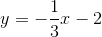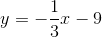Explanation:

Parallel lines have slopes that are opposite reciprocals. Any line perpendicular tomust have a slope of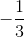.  The only line that doesn't have this slope is, which has a slope of.

### Example Question #22 : How To Find The Equation Of A Perpendicular Line

A vertical line is drawn to connect the x-axis and point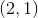.  What must be the equation of the perpendicular line that connects to this point?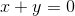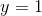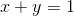Explanation:

The given key word is vertical.  If a perpendicular line that intersects the vertical line, the slope must be zero, and the line would be a horizontal line.  The horizontal line will intersect the point at, which means that the y-value will never change.

The answer is:### Example Question #23 : How To Find The Equation Of A Perpendicular Line

Which of the following is perpendicular to the line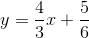?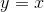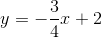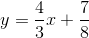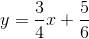Explanation:

First, we want to identify the slope of our given line. In slope-intercept form, a line's slopeis the coefficient ofThe slope of our given line isNow, we can start to find the perpendicular line. Remember that in order for two lines to be perpendicular, their slopes must be negative reciprocals of each other.

In this case, we want to find a line whose slope is the negative reciprocal of. So, we want a line whose slope is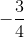With this in mind, the only answer choice with a slope ofisTherefore, the perpendicular line is### Example Question #12 : How To Find The Equation Of A Perpendicular Line

Which of the following equations represents a line that goes through the pointand is perpendicular to the line?Explanation:

In order to solve this problem, we need first to transform the equation from standard form to slope-intercept form:Transform the original equation to find its slope.First, subtractfrom both sides of the equation.Simplify and rearrange.Next, divide both sides of the equation by 6.The slope of our first line is equal to. Perpendicular lines have slopes that are opposite reciprocals of each other; therefore, if the slope of one is x, then the slope of the other is equal to the following:Let's calculate the opposite reciprocal of our slope:The slope of our line is equal to 2. We now have the following partial equation:We are missing the y-intercept,. Substitute the x- and y-values in the given pointto solve for the missing y-intercept.Add 4 to both sides of the equation.Substitute this value into our partial equation to construct the equation of our line:### Example Question #3 : How To Find The Equation Of A Perpendicular Line

What is the equation of a line that runs perpendicular to the line 2x + = 5 and passes through the point (2,7)?

2x – y = 6

x/2 + y = 6

x/2 + y = 5

x/2 – y = 6

2x + y = 7